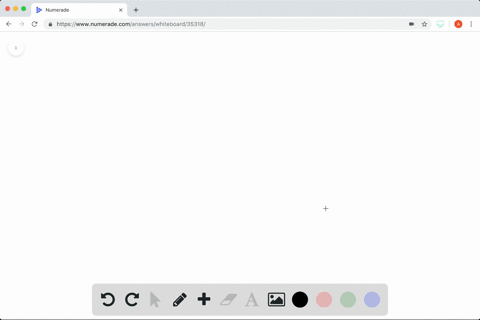Enroll in one of our FREE online STEM summer camps. Space is limited so join now!View Summer Courses### Finding a Particular Solution In Exercises $37-44… 03:26University of Houston Need more help? Fill out this quick form to get professional live tutoring. Get live tutoring Problem 39 Finding a Particular Solution In Exercises$37-44,$find the particular solution of the differential equation that satisfies the initial condition(s).$h^{\prime}(x)=7 x^{6}+5, h(1)=-1\$

$$h(x)=x^{7}+5 x-7$$

## Discussion

You must be signed in to discuss.

## Video Transcript

39. They wanted a toe solve for the initial conditions. So they're given me each crime of X equal seven x to the sick power. Plus I we're a chip one. It was negative. So I'm gonna take my integral, get my original function, plug in my values for my original function and solve for C Take me into Rome. Huge of hex. He won't the integral of seven with six. That's fine. Seven minutes with seven. Your experiment? Yeah, this is really five x to the zero. So when I add on to the rope, get X so us see, I have just next to the seven. X. Now I have to solve for C given eighths of one equals negative one. So when X equals one h, the Becky X equals negative one negative one equals 1227 Sorry. Plus five times one. See negative one. He won't six plus c. Therefore, see who's taking seven. And my answer becomes each of X equals X to the seven. Once my ex minus